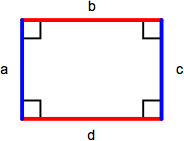## ASVAB Math Knowledge Practice Test 687151

Question 1 of 5
###### Rectangle & Square

A rectangle is a parallelogram containing four right angles. Opposite sides (a = c, b = d) are equal and the perimeter is the sum of the lengths of all sides (a + b + c + d) or, comonly, 2 x length x width. The area of a rectangle is length x width. A square is a rectangle with four equal length sides. The perimeter of a square is 4 x length of one side (4s) and the area is the length of one side squared (s2).If a = c = 5, b = d = 4, what is the area of this rectangle?

 72 18 20 45